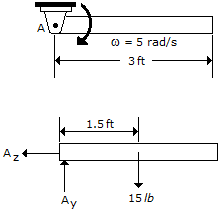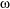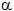# Engineering Mechanics - PKRB: Force and Animation - Discussion

### Discussion :: PKRB: Force and Animation - General Questions (Q.No.8)

8.The 15-lb rod is pinned and has an angular velocity of= 5 rad/s when it is in the horizontal position shown. Determine the rod's angular acceleration and the pin reactions at this instant.

 [A].= 16.1 rad/s2, Ax = 17.47 lb, Ay = 3.75 lb [B].= 64.4 rad/s2, Ax = 17.47 lb, Ay = 30.0 lb [C].= 16.1 rad/s2, Ax = 17.47 lb, Ay = 15.00 lb [D].= 64.4 rad/s2, Ax = 17.47 lb, Ay = 15.00 lb

Explanation:

No answer description available for this question.